Published: 25 October 2022

# The dual-phase-lag bioheat transfer of a skin tissue subjected to thermo-electrical shock

Hamdy M. Youssef1
Raafat A. A. Salem2
1Mechanical Engineering Department-College of Engineering and Islamic Architecture, Umm Al, Qura University, Makkah, Saudi Arabia
2Mathematical Sciences Department, College of Applied Sciences, Umm Al, Qura University, Makkah, Saudi Arabia
Corresponding Author:
Hamdy M. Youssef
Views 93

#### Abstract

The current paper is dealing with the thermal reaction and response of skin tissue subjected to a constant heat flux due to thermo-electrical shock on the bounding plane. The dual-phase-lag bio heat conduction equation based on the Tzou model has been applied and solved in the Laplace transform domain by using a direct method. The numerical inversions of the Laplace transform, and numerical solution has been obtained. The thermos-electrical shock for a small value of time has been considered with constant voltage and resistance. The temperature increment responses have been obtained and discussed with various values of voltage, resistance, and dual-lag value times. Moreover, a comparison between the well-known three models of bioheat conduction of Pennes, Ventott-Cattaneo, and Tzou has been shown in the figure. The results show that the voltage, resistance, and dual-lag value of times have significant effects on the temperature increment distribution and the thermal wave propagation through the skin tissue.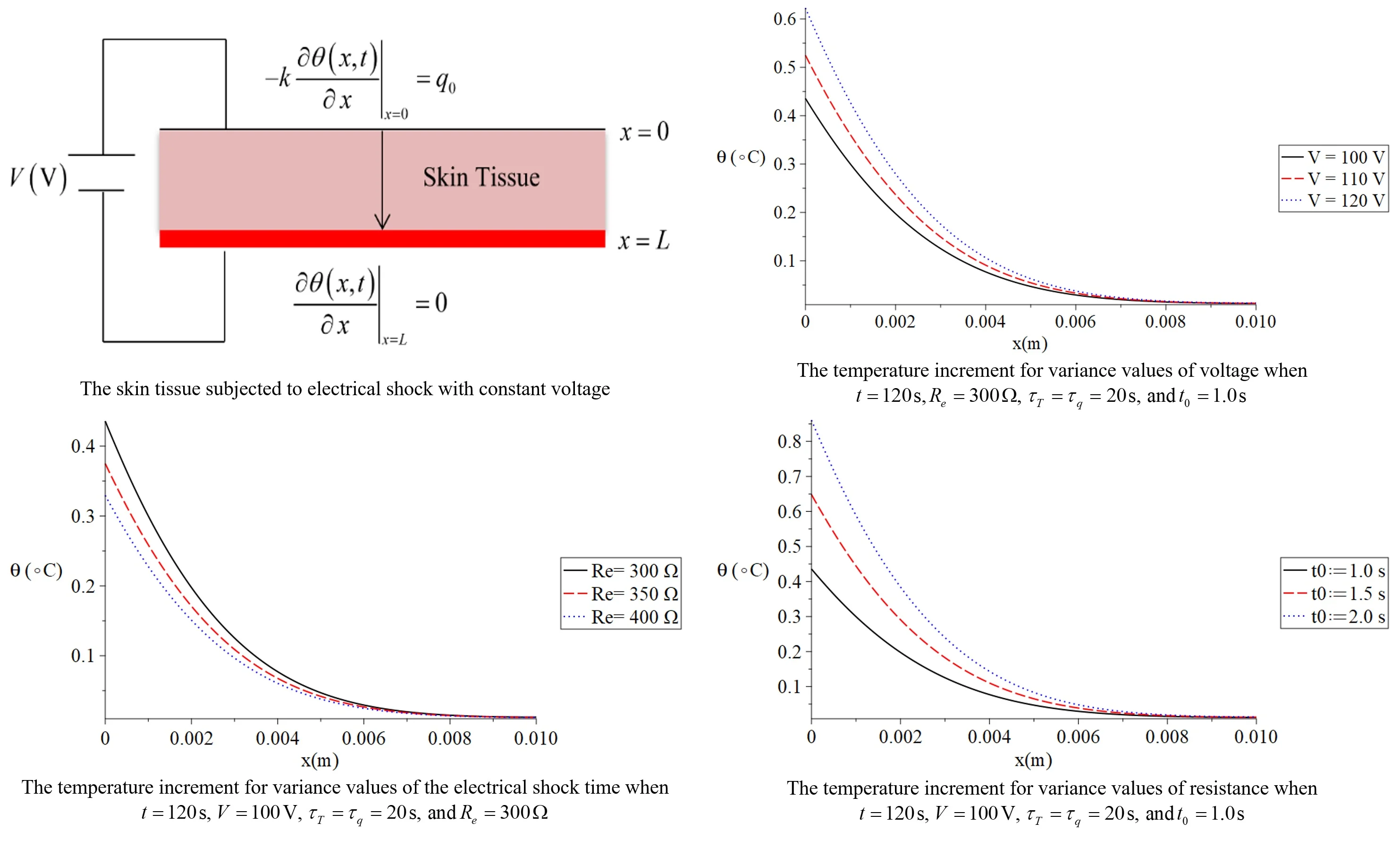#### Highlights

• This research examined how skin tissue responds to a steady heat flux caused by a thermo-electrical shock on the bounding plane in terms of temperature reaction and response.
• A direct technique has been used to apply and solve the dual-phase-lag bio heat conduction equation based on the Tzou model in the domain of the Laplace transform.
• The voltage, resistance, and dual-lag time values significantly affect the distribution of temperature increments and the propagation of thermal waves through skin tissue.
• The Tzou model is the most effective model that accurately predicts the physical behaviour of skin tissue.
• The Tzou and Ventott-Cattaneo models also provide a thermal wave that propagates with a limited speed and is extremely similar to one another.

## 1. Introduction

Many treatments and their applications depend heavily on temperature transmission via live skin tissue. Microwaves, lasers, and other technological advancements have also expanded the field of bioheat transfer work . Researcher Pennes, who improved the parabolic differential equation of the biological tissues for the first bioheat transfer, provides commentary on biothermal transmission analyses in real biological tissues . Additionally, the consistency and nature of thermal activity in biological tissues have been modelled using the Pennes biological transmission approach. Similarities to such erratic outcomes that exhibit non-Fourier or hyperbolic conduction behaviour on the outside. To characterize the kind of hyperbolic equation, Cattaneo and Vernotte gave several modifications of heat conduction of Fourier's law in its linearity form [3, 4]. They proposed a thermal wave model to evaluate the impact of microwave and flux thermal activity. To treat diverse skin tissue disorders without harming the healthy tissue surrounding them, many sorts of treatments have been attempted. The Pennes bioheat transfer equation (PBTE) was analytically solved by Xu et al. They also studied the skin tissue bioheat transmission, skin thermal damage, tissue structure, skin biomechanics, and biothermomechanics  The dual-phase-lag (DPL), parabolic models of biomass transport, and hyperbolic were used to examine the non-Fourier thermomechanical activity of skin tissues in the context of various thermal loading limitations on the surface. Xu and colleagues also observed significant differences between Pennes, thermal waves, and dual-phase-lag (DPL) . Additionally, Rossmann et al. analyzed how biological tissues’ perfusion, thermal characteristics, and dielectric properties change with the temperature at hyperthermic and ablation temperatures . The dual-phase-lag (DPL) approach has been used to expand Tzou’s model while considering the relaxation activity at a high rate of reaction. Despite the slow procedure, the small-scale reaction is detected in time [8, 9]. Tzou introduced a new parameter time of phase lag for a temperature gradient . Dual-phase-lag (DPL) heat conduction model has been used by Askarizadeh et al. to address transient heat transmission issues in skin tissue .

Before analyzing the general bioheat transfer paradigm in live tissue, Liu et al. . The two-stage model for heat transmission issues in biological tissue uses the hyperthermia process to assess Liu et al. [13, 14]. The Liu and Chen thermal conductor model (DPL) employed non-Fourier thermal activity to characterize the thermal transit taking place in biological tissue and to diagnose hyperthermia .

Liu and Xu developed a closed-form analytical method to solve the Pennes bioheat equation for fluctuations in skin tissue temperature caused by sinusoidal heat flow . Ahmadika et al. will have the analytical solution for the Pennes equations’ bioheat (parabolize) and hyperbolized bioheat transfer models for periodic constant and pulsed train heat flow boundary conditions . Youssef and Alghamdi investigated a one-dimensional application of thermoelastic dual-phase-lag (DPL) in skin tissue under specific heat stress .

The body resists the flow of current. The skin is where the body experiences more than 99 % of its total electrical resistance. Ohms are used to measuring resistance. A calloused, dry hand may contain more than 100,000 due to the stratum corneum’s thick outer layer of dead cells. Due to the damp, moderately salty tissues beneath the skin, the internal body resistance is around 300. If there is skin breakdown due to high voltage, a cut, a severe abrasion, or submersion in water, the skin resistance can be efficiently overcome [19-21]. The skin behaves similarly to a capacitor or other electrical device in that it permits greater current to pass when voltage changes quickly. If one's hand is holding a metal instrument that abruptly comes into contact with a source of voltage, a quickly varying voltage will be applied to the palm and fingers. This kind of interaction will result in a substantially higher body current amplitude than would otherwise happen [19-21].

A quick, intense reaction to an electric current flowing through any portion of a person’s body is known as an electric shock. Electric shock results in electrocution, which is death. Tissue damage caused by electrical current, or voltage is referred to as primary electrical injury. Falls are a frequent source of secondary injuries. High resistance in the skin’s outer layer disintegrates above 500 V or greater. This significantly reduces the body’s resistance to current flow [19-21]. As a result, the quantity of current that flows at any given voltage increases. Skin breakdown can occasionally appear as tiny wounds the size of a pinhead that is simple to ignore. They frequently indicate that a significant quantity of current could enter the body. Muscles, nerves, and other tissues may sustain profound tissue damage because of this current. This is one of the reasons why high-voltage accidents frequently result in considerable deep tissue harm but little surface burns [19-21]. Youssef and El-Bary studied the thermal damage of the tumour tissue due to the thermoelectrical effect based on the Thomson effect. Different voltage and resistance effects have been studied and discussed in this work for three different types of tumour tissues .

The present paper’s goal is to build a mathematical model of bioheat skin tissue within the framework of a dual-phase-lag heat conduction model (Tzou model). The skin tissue’s surface is treated with an electrical shock with a constant voltage for a short period. The work is unique in that it investigates the impacts of dual-phase-lag time parameters, voltage value, and skin resistance on the temperature increment distribution using three distinct bioheat models: Pennes, Ventott-Cattaneo, and Tzou.

### 1.1. Pennes model of bioheat transfer for biological tissues

The bioheat transmission model was developed to examine the time-dependent temperature change as a heat response generated by either a heat source or thermal heating. Pennes developed the first model of biological tissues based on Fourier's law of heat conduction, which is as follows [18, 23, 24]:

1
$K{\nabla }^{2}T=\rho C\frac{\partial T}{\partial t}-{\rho }_{b}{w}_{b}{C}_{b}\left({T}_{b}-T\right)-\left({Q}_{met}+{Q}_{ext}\right),$

where $\rho$, $C$ and $K$ are the density, specific heat, and thermal conductivity of the tissue, respectively. ${C}_{b}$, ${w}_{b}$, ${\rho }_{b}$ and ${T}_{b}$ denote the specific heat of the blood, blood perfusion rate, blood density, and blood temperature, respectively. $T$ is the absolute temperature function. ${Q}_{met}$ denotes the metabolic heat generated by the chemical reaction inside the tissue, and it is constant, and the external heat source is given by ${Q}_{ext}$. Moreover, ${\nabla }^{2}$ is the Laplace operator.

### 1.2. The Ventott-Cattaneo model of bioheat conduction (Modified Fourier law)

Ventott-Cattaneo (V-C) amended the conventional Fourier thermal drive law by postulating the assumption of limited thermal wave propagation speed, resulting in the following hyperbolic form of the thermal wave [18, 23, 24]:

2
$K{\nabla }^{2}T=\rho C\left(1+{\tau }_{q}\frac{\partial }{\partial t}\right)\frac{\partial T}{\partial t}+{w}_{b}{C}_{b}{\rho }_{b}\left(1+{\tau }_{q}\frac{\partial }{\partial t}\right)\left(T-{T}_{b}\right)$
$-\left(1+{\tau }_{q}\frac{\partial }{\partial t}\right)\left({Q}_{met}+{Q}_{ext}\right),$

where ${\tau }_{q}=\frac{\alpha }{{c}_{0}^{2}}>0$ is a material property and is called the relaxation time parameter, and $\alpha$ is the thermal diffusivity while ${c}_{0}$ is the speed of the thermal wave inside the medium.

### 1.3. Dual-phase-lag model (DPL) of bioheat transfer (Tzou model)

The dual-phase-lag (DPL) model based on the dual reaction between the gradient of the temperature $\nabla T$ and the heat flux $q$ has been modified by Tzou to be in the following heat conduction equation [18, 23, 24]:

3
$K\left(1+{\tau }_{T}\frac{\partial }{\partial t}\right){\nabla }^{2}T=\rho C\left(1+{\tau }_{q}\frac{\partial }{\partial t}\right)\frac{\partial T}{\partial t}+{w}_{b}{C}_{b}{\rho }_{b}\left(1+{\tau }_{q}\frac{\partial }{\partial t}\right)\left(T-{T}_{b}\right)$
$-\left(1+{\tau }_{q}\frac{\partial }{\partial t}\right)\left({Q}_{met}+{Q}_{ext}\right),$

where ${\tau }_{T}\ge 0$ is the second parameter of the relaxation time, which is the phase lag of the temperature gradient passing through the medium.

We assume the temperature increment as follows:

4
$\theta =T-{T}_{b}.$

Hence, we have:

5
$K\left(1+{\tau }_{T}\frac{\partial }{\partial t}\right){\nabla }^{2}\theta =\rho C\left(1+{\tau }_{q}\frac{\partial }{\partial t}\right)\frac{\partial \theta }{\partial t}+{w}_{b}{C}_{b}{\rho }_{b}\left(1+{\tau }_{q}\frac{\partial }{\partial t}\right)\theta$
$-\left(1+{\tau }_{q}\frac{\partial }{\partial t}\right)\left({Q}_{met}+{Q}_{ext}\right).$

## 2. Basic formulation

We proposed the medium $0\le x\le L$ has been filled with skin tissue as in figure 1 and obeys the dual-phase-lag (DPL) as in Eq. (5) [18, 24].

Initially, the medium is dormant and has no external heat source $\left({Q}_{ext}=0\right)$while the metabolic heat source ${Q}_{met}$ is constant. The bounding plane of the outer surface of the skin tissue $x=0$ is subjected to constant heat flux ${q}_{0}$, while the bounding plane of the inner surface $x=L$ has no heat flux (see Fig. 1).

Hence, the heat conduction equations take the forms:

6
$\left({\tau }_{T}\frac{\partial }{\partial t}+1\right)\frac{{\partial }^{2}\theta }{\partial {x}^{2}}=\left(\frac{{\tau }_{q}\rho C}{K}\frac{{\partial }^{2}}{\partial {t}^{2}}+\frac{\left(\rho C+{\tau }_{q}{w}_{b}{C}_{b}{\rho }_{b}\right)}{K}\frac{\partial }{\partial t}+\frac{{w}_{b}{C}_{b}{\rho }_{b}}{K}\right)\theta -\frac{{Q}_{met}}{K}.$

Fig. 1The skin tissue subjected to electrical shock with constant voltage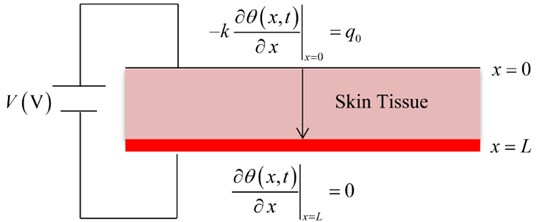The initial conditions are:

7
${\theta \left(x,t\right)|}_{t=0}={\frac{\partial \theta \left(x,t\right)}{\partial t}|}_{t=0}=0.$

The boundary conditions are:

8
${\frac{\partial \theta \left(x,t\right)}{\partial x}|}_{x=0}=-\frac{{q}_{0}}{K},{\frac{\partial \theta \left(x,t\right)}{\partial x}|}_{x=L}=0.$

The solution in the Laplace transform domain:

9
$\stackrel{-}{F}\left(x,s\right)=L\left(f\left(x,t\right)\right)={\int }_{0}^{\infty }f\left(x,t\right){e}^{-st}dt,t\ge 0,$

where the inverse of the Laplace transform takes the form:

10
${L}^{-1}\left(\stackrel{-}{f}\left(s\right)\right)=f\left(t\right)\approx \frac{{e}^{\kappa t}}{t}\left[\frac{1}{2}\stackrel{-}{f}\left(\kappa \right)+\mathrm{R}\mathrm{e}\sum _{n=1}^{N}{\left(-1\right)}^{n}\stackrel{-}{f}\left(\kappa +\frac{in\pi }{t}\right)\right],$

where $\mathrm{R}\mathrm{e}$ means the real part, while $i$ is the imaginary number unit, for faster convergence, many experiments confirmed that the value of the parameter $\kappa$ could satisfy the relation $\kappa t\approx$ 4.7 [8, 25].

Then, we have:

11
$\frac{{d}^{2}\stackrel{-}{\theta }\left(x,s\right)}{d{x}^{2}}-{\lambda }^{2}\left(s\right)\stackrel{-}{\theta }\left(x,s\right)=-\gamma \left(s\right),$

where:

12
${\lambda }^{2}\left(s\right)=\frac{{\tau }_{q}\rho C{s}^{2}+\left(\rho C+{\tau }_{q}{w}_{b}{C}_{b}{\rho }_{b}\right)s+{w}_{b}{C}_{b}{\rho }_{b}}{\left({\tau }_{T}s+1\right)K},\gamma \left(s\right)=\frac{{Q}_{met}}{sK},$
${\frac{d\stackrel{-}{\theta }\left(x,s\right)}{dx}|}_{x=0}=-\frac{{q}_{0}}{sK},{\frac{d\stackrel{-}{\theta }\left(x,s\right)}{dx}|}_{x=L}=0.$

The skin tissue is subjected to thermal shock that comes from the thermal effect due to electrical shock with constant voltage (Joule’s equation of electrical heating) $V\left(V\right)$ and constant resistance ${R}_{e}\left(\mathrm{\Omega }\right)$ when the current has a vibration of 60 Hz, the mean value of the skin resistance will be considered in the range 300-400 Ω [7, 19-22].

Then, Joule’s equation of electrical heating is given by [7, 19, 21, 22]:

13
${q}_{0}=\frac{{V}^{2}{t}_{0}}{{R}_{e}},$

where ${t}_{0}\left({t}_{0}>0\right)$ is a parameter which gives the time interval of the electrical shock.

The general solution of the Eq. (11) is given by:

14
$\stackrel{-}{\theta }\left(x,s\right)={\theta }_{1}\left(s\right){e}^{-\lambda x}+{\theta }_{2}\left(s\right){e}^{\lambda x}+\frac{\gamma \left(s\right)}{{\lambda }^{2}\left(s\right)},$

where ${\theta }_{1}\left(s\right)$ and ${\theta }_{2}\left(s\right)$ are some parameters which must be determined by using the boundary condition Eq. (12).

Hence, we have:

15
$-{\theta }_{1}+{\theta }_{2}=-\alpha ,-{\theta }_{1}{e}^{-\lambda L}+{\theta }_{2}{e}^{\lambda L}=0,$

where $\alpha ={q}_{0}/sK\lambda$.

By solving the above system, we obtain:

16
${\theta }_{1}=\frac{\alpha {e}^{\lambda L}}{{e}^{\lambda L}-{e}^{-\lambda L}},{\theta }_{2}=\frac{\alpha {e}^{-\lambda L}}{{e}^{\lambda L}-{e}^{-\lambda L}}.$

Then, we have the solution in the form:

17
$\stackrel{-}{\theta }\left(x,s\right)=\frac{\alpha \mathrm{c}\mathrm{o}\mathrm{s}\mathrm{h}\left(\lambda \left(L-x\right)\right)}{\mathrm{s}\mathrm{i}\mathrm{n}\mathrm{h}\left(L\lambda \right)}+\frac{\gamma }{{\lambda }^{2}},$

which completes the solution in the Laplace transform domain where the solution in the physical time domain can be obtained by applying iteration Eq. (10).

## 3. Numerical results

The temperature distribution through skin tissue is explored in this work using three bioheat transfer models (Pennes, Ventott-Cattaneo, and Tzou) under constant heat flow circumstances on the skin surface. Table 1 shows the values of the essential thermal parameters employed in the current computations [5, 18, 23, 24, 26].

Table 1The material properties of the skin tissue

 Parameter Value Unit Parameter Value Unit $\mathrm{K}$ 0.215 W/m°C ${\mathrm{W}}_{\mathrm{b}}$ 0.00052 ml/C m $\mathrm{\rho }$ 1000 kg/m3 ${\mathrm{T}}_{\mathrm{b}}$ 37 °C ${\mathrm{\rho }}_{\mathrm{b}}$ 1060 kg/m3 ${\mathrm{\tau }}_{\mathrm{T}},{\mathrm{\tau }}_{\mathrm{q}}$ 20.0 s $\mathrm{C}$ 4187 J/kg°C ${\mathrm{t}}_{0}$ 1.0 s ${\mathrm{C}}_{\mathrm{b}}$ 3800 J/kg°C $L$ 0.01 m

## 4. Discussions

Fig. 2 shows the temperature increment distribution for variance values of voltage $V=$ (100, 110, 120) V when $t=$ 120 s, ${R}_{e}=$ 300 Ω, ${\tau }_{T}={\tau }_{q}=$ 20 s, and ${t}_{0}=$ 1.0 s. It is noted that the value of the voltage of the thermo-electrical shock has a significant effect on the thermal wave. The value of the temperature increment increases when the value of the voltage increases. The values of the temperature increment on the boundary of the skin tissue $x=0$ take the following order:

18
${\theta \left(V=100\mathrm{V}\right)|}_{x=0}<{\theta \left(V=110\mathrm{V}\right)|}_{x=0}<{\theta \left(V=120\mathrm{V}\right)|}_{x=0}.$

Fig. 3 shows the temperature increment distribution for variance values of skin tissue resistance ${R}_{e}=$(300, 350, 400) Ω when $t=$ 120 s, $V=$ 100 V, ${\tau }_{T}={\tau }_{q}=$20 s, and ${t}_{0}=$ 1.0 s. It is noted that the value of the voltage of the skin tissue resistance has a significant effect on the thermal wave. The value of the temperature increment decreases when the value of the skin tissue resistance increases. The values of the temperature increment on the boundary of the skin tissue $x=0$ take the following order:

19
${\theta \left({R}_{e}=400\mathrm{\Omega }\right)|}_{x=0}<{\theta \left({R}_{e}=350\mathrm{\Omega }\right)|}_{x=0}<{\theta \left({R}_{e}=300\mathrm{\Omega }\right)|}_{x=0}.$

Fig. 2The temperature increment for variance values of voltage when t= 120 s, Re= 300 Ω, τT=τq= 20 s, and t0= 1.0 s.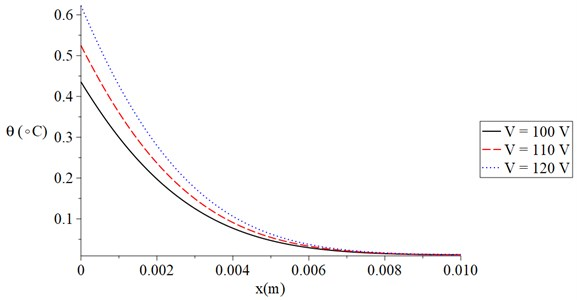Fig. 3The temperature increment for variance values of resistance when t= 120 s, V= 100 V, τT=τq= 20 s, and t0= 1.0 s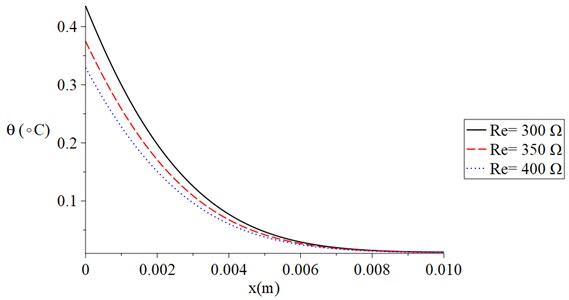Fig. 4 shows the temperature increment distribution for variance values of electrical shock time ${t}_{0}=$(1.0, 1.5, 2.0) s when $t=$ 120 s, $V=$ 100 V, ${\tau }_{T}={\tau }_{q}=$ 20 s, and ${R}_{e}=$ 300 Ω. It is noted that the value of the electrical shock time has a significant effect on the thermal wave. The value of the temperature increment increases when the value of the electrical shock time increases. The values of the temperature increment on the boundary of the skin tissue $x=0$ take the following order:

20
${\theta \left({t}_{0}=1.0\mathrm{s}\right)|}_{x=0}<{\theta \left({t}_{0}=1.5\mathrm{s}\right)|}_{x=0}<{\theta \left({t}_{0}=2.0\mathrm{s}\right)|}_{x=0}.$

Fig. 5 shows the temperature increment distribution for variance situations of the dual-lag times parameters $\left({\tau }_{q}<{\tau }_{T},{\tau }_{q}={\tau }_{T},{\tau }_{q}>{\tau }_{T}\right)$ when $t=$ 120 s, $V=$ 100 V, ${t}_{0}=$ 1.0 s and ${R}_{e}=$ 300 Ω. It is noted that the value of the dual-lag times parameters has a significant effect on the thermal wave. The values of the temperature increment on the boundary of the skin tissue $x=0$ take the following order:

21
${\theta \left({\tau }_{T}<{\tau }_{q}\right)|}_{x=0}<{\theta \left({\tau }_{T}={\tau }_{q}\right)|}_{x=0}<{\theta \left({\tau }_{T}>{\tau }_{q}\right)|}_{x=0}.$

We can see that the situation when the value of the lag-time of the temperature is smaller than the value of the lag-time parameter of the temperature gradient, generates a thermal wave which has a finite speed of propagation where in this case, the temperature increment goes to zero before the other two cases.

Fig. 4The temperature increment for variance values of the electrical shock time when t= 120 s, V= 100 V, τT=τq= 20 s, and Re= 300 Ω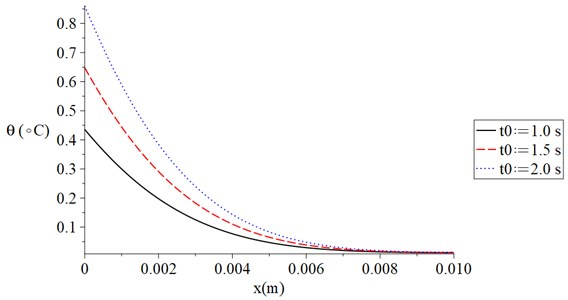Fig. 5The temperature increment for variance situations of the dual-lag times parameters when t= 120 s, V= 100 V, t0= 1.0 s and Re= 300 Ω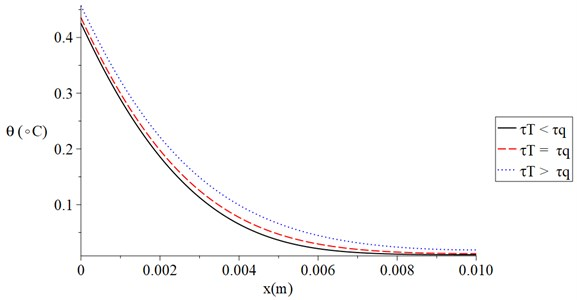Fig. 6 shows the temperature increment distribution for Pennes, Ventott-Cattaneo, and Tzou models when $t=$ 120 s, $V=$ 100 V, ${t}_{0}=$ 1.0 s, and ${R}_{e}=$ 300 Ω. It is noted that the temperature increment distribution has different values based on the three different models. The values of the temperature increment take the following order:

22
$\theta \left(\mathrm{T}\mathrm{z}\mathrm{o}\mathrm{u}\right)<\theta \left(\mathrm{V}\mathrm{e}\mathrm{n}\mathrm{t}\mathrm{o}\mathrm{t}\mathrm{t}-\mathrm{C}\mathrm{a}\mathrm{t}\mathrm{t}\mathrm{a}\mathrm{n}\mathrm{e}\mathrm{o}\right)<\theta \left(\mathrm{P}\mathrm{e}\mathrm{n}\mathrm{n}\mathrm{e}\mathrm{s}\right).$

The results of this figure proved that the Tzou model is the most successful model which agrees with the physical behaviour of the skin tissue. Moreover, Tzou and Ventott-Cattaneo models are very close and offer a thermal wave propagating with a finite speed.

Fig. 6The temperature increment for Pennes, Ventott-Cattaneo, and Tzou models when t= 120 s, V= 100 V, t0= 1.0 s, and Re= 300 Ω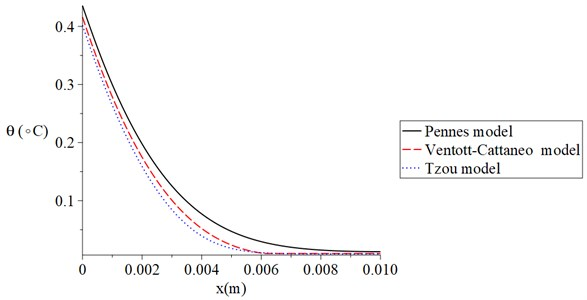## 5. Conclusions

This research examined how skin tissue responds to a steady heat flux caused by a thermo-electrical shock on the bounding plane in terms of temperature reaction and response. A direct technique has been used to apply and solve the dual-phase-lag bio heat conduction equation based on the Tzou model in the domain of the Laplace transform. The Laplace transforms’ numerical inversions and solutions have been discovered. With constant voltage and resistance, the thermos-electrical shock for a short period has been explored.

With different voltage, resistance, and dual-lag time values, the temperature increment responses have been measured and discussed. Additionally, a comparison of the well-known Pennes, Ventott-Cattaneo, and Tzou bioheat conduction models has been illustrated.

The findings demonstrate that the voltage, resistance, and dual-lag time values significantly affect the distribution of temperature increments and the propagation of thermal waves through skin tissue.

The outcomes of this research demonstrated that the Tzou model is the most effective model that accurately predicts the physical behaviour of skin tissue. The Tzou and Ventott-Cattaneo models also provide a thermal wave that propagates with a limited speed and is extremely similar to one another.

#### Acknowledgements

The authors have not disclosed any funding.

#### Cited by

Thermo-Electrical Influence of Graphene Nano-Strip on Viscothermoelastic Nanobeam Vibration